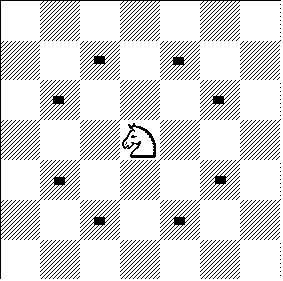## Problem D: Knight's TripIn chess, each move of a knight consists of moving by two squares horizontally and one square vertically, or by one square horizontally and two squares vertically. A knight making one move from location (0,0) of an infinite chess board would end up at one of the following eight locations: (1,2), (-1,2), (1,-2), (-1,-2), (2,1), (-2,1), (2,-1), (-2,-1).

Starting from location (0,0), what is the minimum number of moves required for a knight to get to some other arbitrary location (x,y)?

### Input Specification

Each line of input contains two integers x and y, each with absolute value at most one billion. The integers designate a location (x,y) on the infinite chess board. The final line contains the word END.

```1 2
2 4
END
```

### Output Specification

For each location in the input, output a line containing one integer, the minimum number of moves required for a knight to move from (0,0) to (x, y).

```1
2
```

Ondřej Lhoták